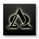2808 lượt xem
Smoothed version, experimental.
study(title="[RS]Murrey's Math Lines Smooth Channel", shorttitle="[RS]MMLSC", overlay=true)
length00 = input(100)
fmultiplier = input(defval=0.125, type=float)
smooth = input(1)
hhi = highest(high, length00)
llo = lowest(low, length00)
fraction = sma((hhi - llo) * fmultiplier, smooth)
midline = sma((llo + hhi) / 2, smooth)
plot(midline, color=gray, linewidth=3)
plot(midline + fraction * 1, color=gray)
plot(midline + fraction * 2, color=gray)
p00 = plot(midline + fraction * 3, color=gray, linewidth=1)
p01 = plot(midline + fraction * 4, color=gray, linewidth=2)
plot(midline + fraction * 5, color=gray)
plot(midline + fraction * 6, color=gray)

plot(midline - fraction * 1, color=gray)
plot(midline - fraction * 2, color=gray)
p02 = plot(midline - fraction * 3, color=gray, linewidth=1)
p03 = plot(midline - fraction * 4, color=gray, linewidth=2)
plot(midline - fraction * 5, color=gray)
plot(midline - fraction * 6, color=gray)

fill(p00, p01, color=gray, transp=50)
fill(p02, p03, color=gray, transp=50)

## Bình luậnGood discussion. For comparison purposes, are any of us capable of converting Richard's script to a horizontal line of the 8 levels +/- the 2 levels into horizontal levels that are set on the range of the prior 5 day or 20 day period? We could overlay that on his existing script to get a "grid" to compare to other grids of fib level, fib channels, pivot levels ... I am suspecting the horizontal version will have interesting confluence points but I cannot figure how to script it. To be clear, the needed script would be a supplement to what Richard has made and an overlay for it.
Phản hồiHere is a basic system that can prove to be very useful using Ricardo's Murrey Math indicators. It is based on the crossover of a Median line, short over the long periods selected by the user. This chart I changed up a bit from the prior posting to clean it up. Short period of 24 vs 144. Check out the chart notations, thats all you need and you can trade this all week by picking out value in the price divergences. When both period extreme zones are entered by price, these are great areas of value to. I did add a time element as well. I took a Fib Channel and it requires three points. I turned it into a Fib Time extension by starting at the beginning of the Weekly pivot, sought a touch of the first support or resistance 1, in this case it was a touch of S1. Then drew back to the beginning of that S1 line, creates a channel which allows colorization between the zones, very helpful visually to me. Notice how fib time barriers and the convergence of MTF zones has a heavy influence on price.

Phản hồicooney_s
sheesh edit #2: fib channel is drawn as such; Beginning of weekly pivot, beginning of S1 pivot (since that is the first support or resistance pivot touched) THEN over to where price touched the S1. Sorry about the confusion. The rest is all good.
Phản hồicooney_s
You reinvented ichimoku coondawg. :)
Phản hồiIvanLabrie
thanks, just add it to the toolbox!
Phản hồijackvmk
Thank you. Just showing some use for another great tool the awesome TV sciptors post.
Phản hồi